# Strong colorability

 Importance: High ✭✭✭
 Author(s): Aharoni, Ron Alon, Noga Haxell, Penny E.
 Subject: Graph Theory » Coloring » » Vertex coloring
 Keywords: strong coloring
 Posted by: berger on: March 27th, 2007

Letbe a positive integer. We say that a graphis strongly-colorable if for every partition of the vertices to sets of size at mostthere is a proper-coloring ofin which the vertices in each set of the partition have distinct colors.

Conjecture   Ifis the maximal degree of a graph, thenis strongly-colorable.

Haxell proved that ifis the maximal degree of a graph, thenis strongly-colorable. She later proved that the strong chromatic number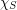is at mostfor sufficiently largedepending on. Aharoni, Berger, and Ziv proved the fractional relaxation.

### Recent progress

Haxell proved that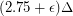is sufficient for sufficiently largedepending on. (JGT, 2008)

Aharoni, Berger, and Ziv proved the fractional relaxation, i.e. that with partition cliques of size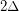we have a fractionalcolouring. (Combinatorica, 2007)

### Problem Solved!

See "On the Strong Chromatic Number of Graphs" by Maria Axenovich and Ryan Martin (2006)

### only partly solved

The paper cited (available at http://orion.math.iastate.edu/axenovic/Papers/Martin_Strong.pdf) only resolves the above conjecture for graphs G which have maximum degree at least |V(G)|/6.

### That theorem has been proved

That theorem has been proved in an even older paper by another set of authors.

After a quick search I found that paper at http://abel.math.umu.se/~klasm/Uppsatser/factor.pdf

### still only a partial solution

Once again, the paper cited offers only a partial solution. Quoting from the paper, "From Theorem 1.1, we conclude that the strong chromatic number can be bounded byif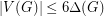. This result should not be compared with the more complete and difficult result of Alon." Here, the difficult result of Alon is the theorem that there exists a fixed constantso that every graph of maximum degreeis strongly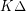-colorable.. precisely the result which is sharpened by this conjecture.